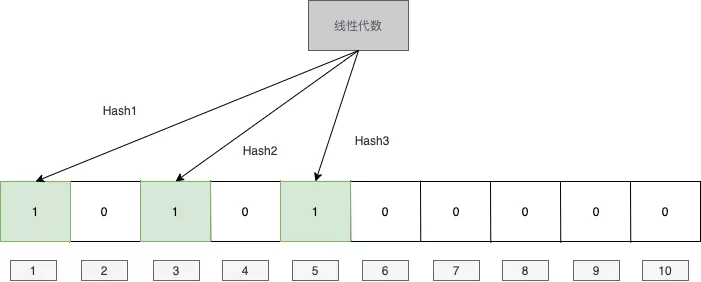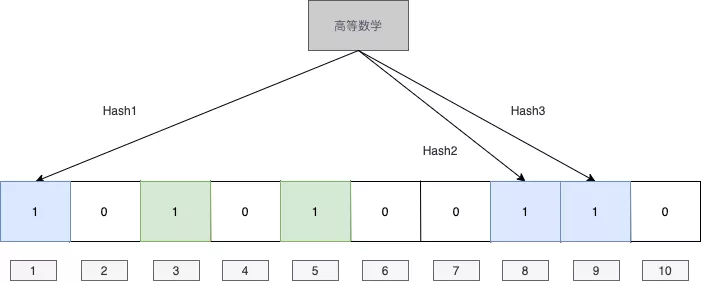## # 原理## # 代码

``````
def BloomFilter(filter, value, hash_functions):
m = len(filter)
for func in hash_functions:
idx = func(value) % m
filter[idx] = True
return filter

def MemberInFilter(filter, value, hash_functions):
m = len(filter)
for func in hash_functions:
idx = func(value) % m
if not filter[idx]:
return False
return True
``````

## # 错误率计算

f(k)=(1−b−k)kln⁡f(k)=kln⁡(1−b−k)

1f(k)f′(k)=ln(1−b−k)+kb−kln⁡b1−b−k

ln⁡(1−b−k)(1−b−k)=−kb−kln⁡bln⁡(1−b−k)(1−b−k)=b−kln⁡b−k1−b−k=b−kb−k=12

## # 原文链接

https://www.cnblogs.com/techflow/p/12310669.html